## Machine Learning in the Browser with Deep Neural Networks

### A Visual Introduction

#### http://js-kongress.de 2016

http://bit.ly/ml-js-kongress

### What is machine learning?https://twitter.com/DJCordhose/status/785108341823594496

### Applications of machine learning?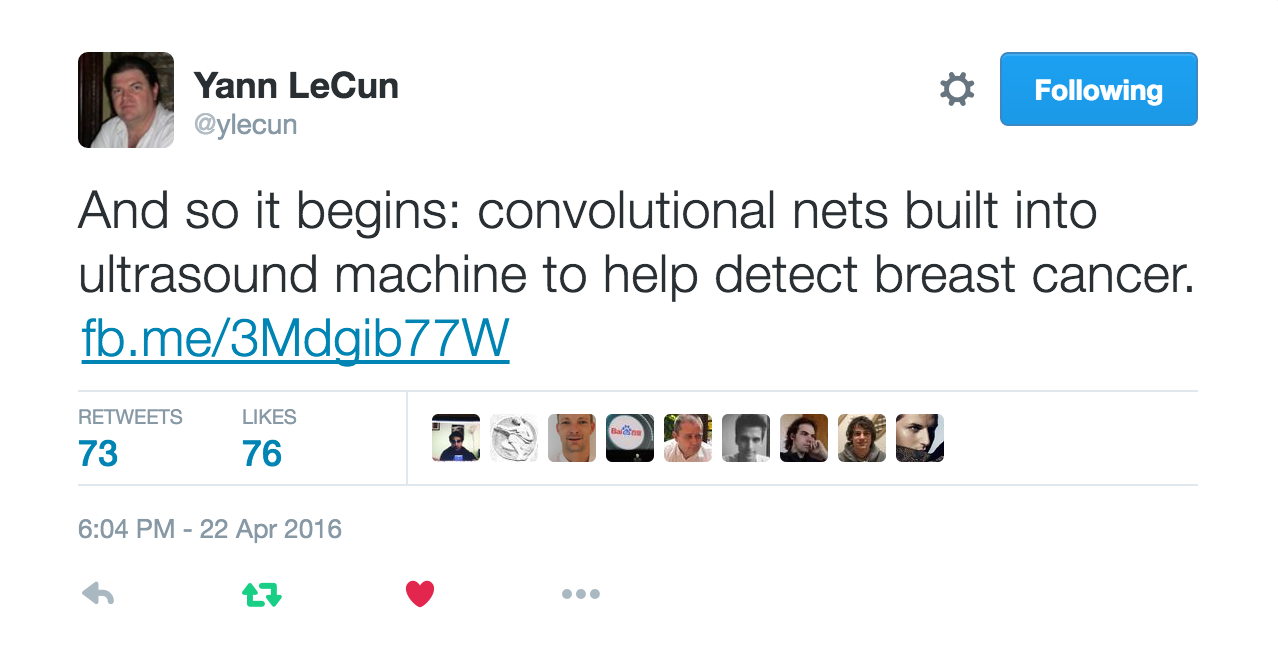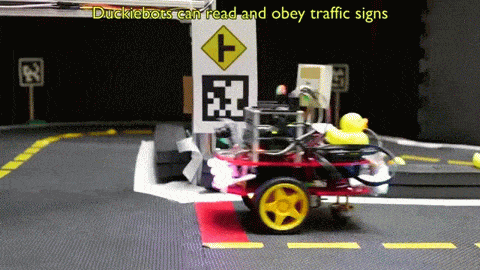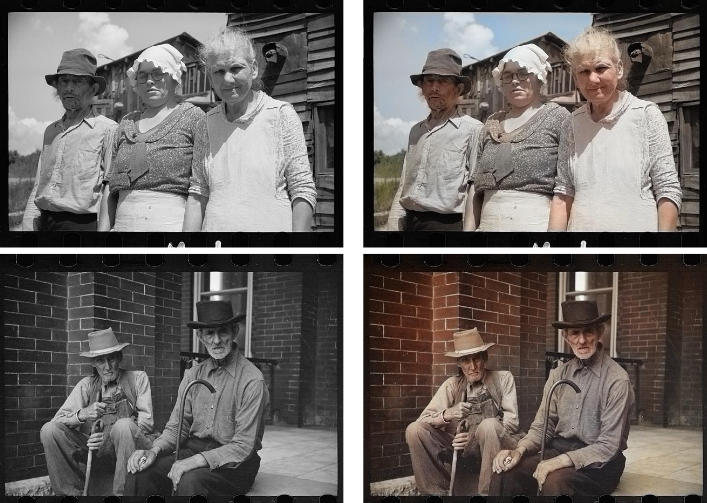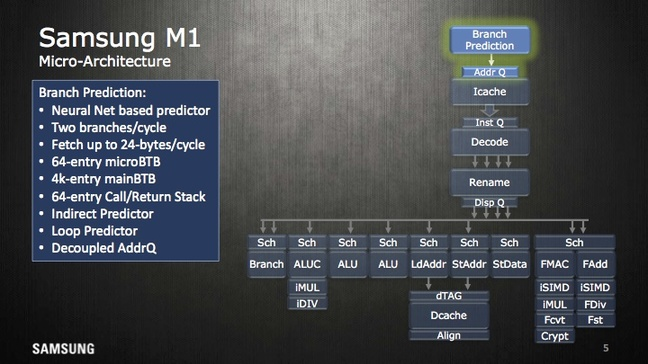### Why JavaScript for Machine Learning?

• Python and R are predominant
• Have a large and mature set of libs
• Are reasonably fast
• Using binding to C/C++ or Fortran
• JavaScript has benefits, though
• might be the language you are most comfortable with
• might be the only language around
• because all you have is a browser
• e.g. AI in browser based game
• zero installation, easy to get started
• combination with interactive visualizations

## But for today: Introducing Deep Neural Networks

### The perceptron - where it all beginshttp://www.theprojectspot.com/tutorial-post/introduction-to-artificial-neural-networks-part-1/7

### Implementing it in pure JavaScript

``````
// Initial weights
let w0 = 3, w1 = -4, w2 = 2;
``````
``````
function perceptron(x1, x2) {
const sum = w0 + w1 * x1 + w2 * x2;
return activation(sum);
}
``````
``````
function activation(z) {
// in this case a sigmoid function (alt.: tanh, linear, relu)
return 1 / (1 + Math.exp(z * -1));
}
``````### Visualizing what a neuron can do

can emulate most logic functions (NOT, AND, OR, NAND)

• output separates plane into two regions using a line
• such regions are called linearly separable
• can be trained by adjusting weights of inputs based on error in output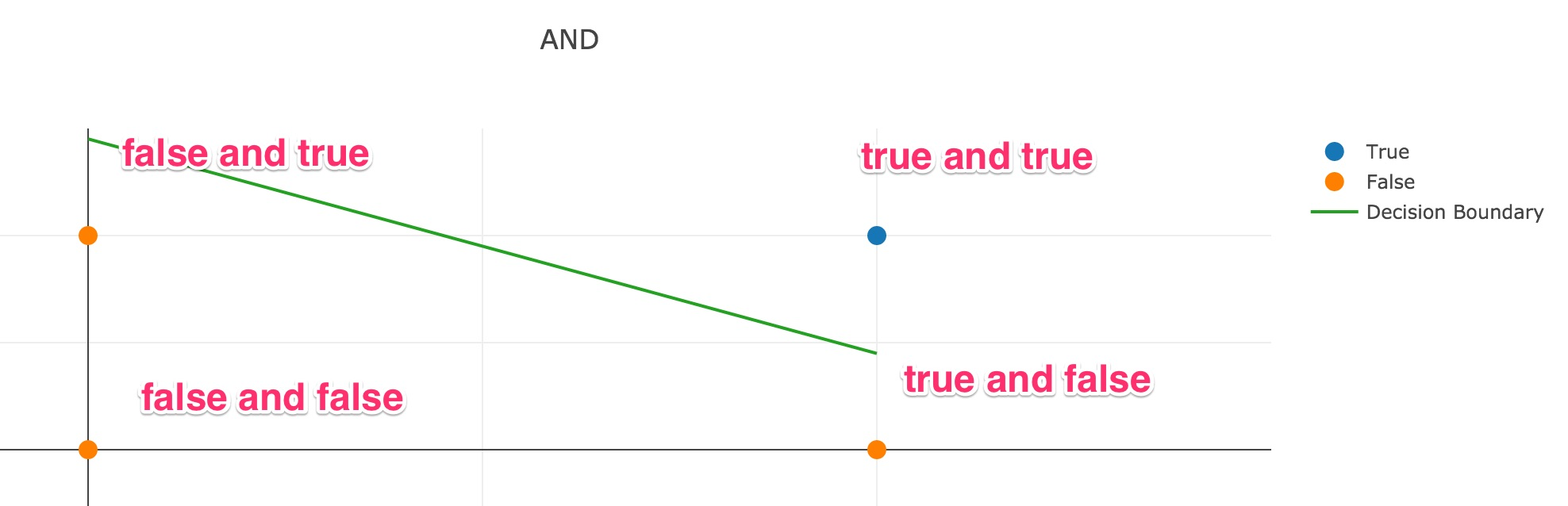percepton training visualization (initial version provided as a courtesy of Jed Borovik)

### XOR - What a neuron can NOT do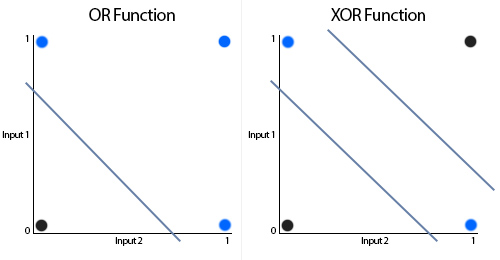http://www.theprojectspot.com/tutorial-post/introduction-to-artificial-neural-networks-part-1/7

Because it would require two lines for separation

### Introducing Feedforward Neural Networks

Arraging many Neurons in layers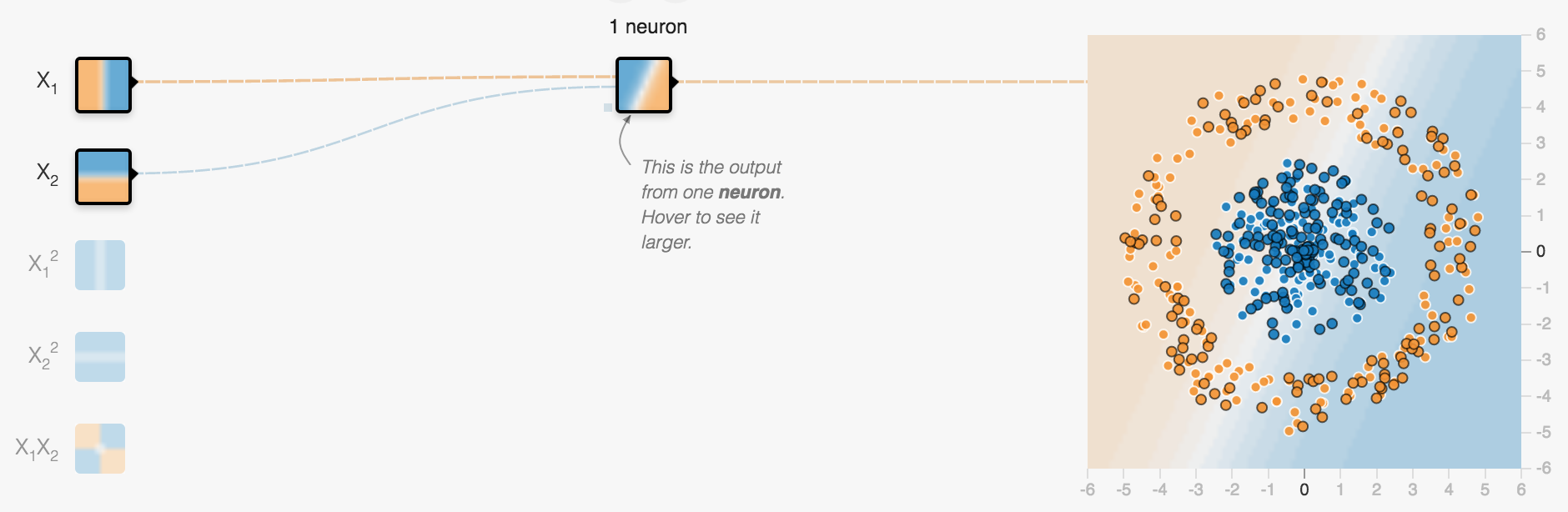Using the Tensorflow Playground

### Introducing our classification example

• dots are placed on a plane with a certain pattern
• two different kinds (either blue or orange)
• network shall learn that pattern
• uses a set of known dots for training
• then make predictions for dots it has not seen before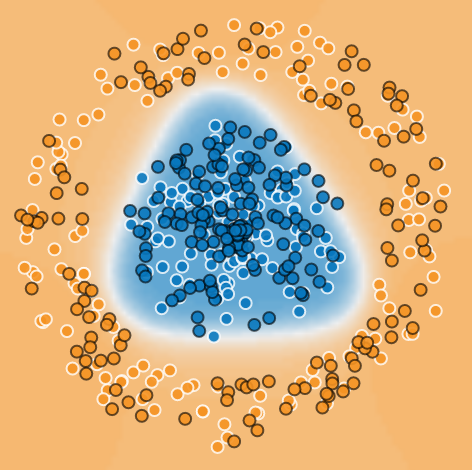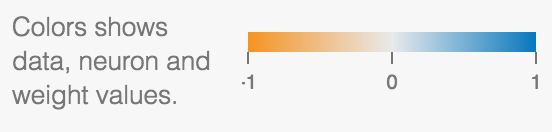## Is classification at all relevant?

Why would I care how to tell blue from organge spots?

### First layers takes inputs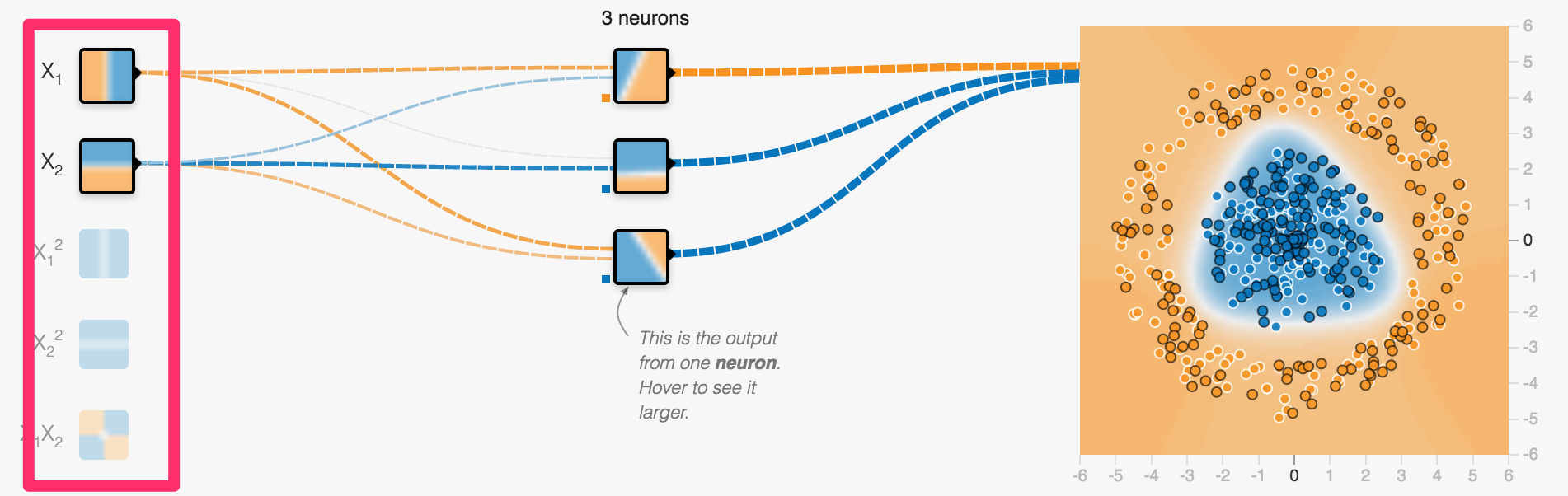x and y coordinate of a spot in our example

### Middle layer(s) called hidden layer(s)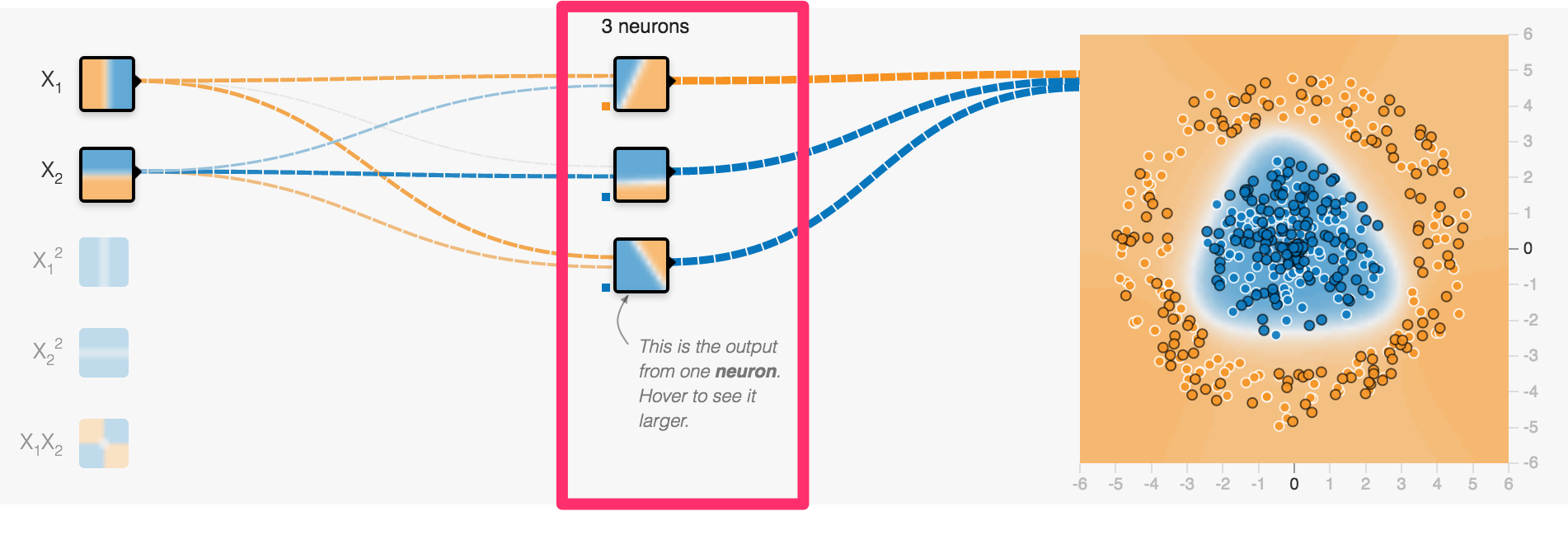Just a single layer containing 3 neurons

### Output layer creates output

in our example using a single neuron, tanh activation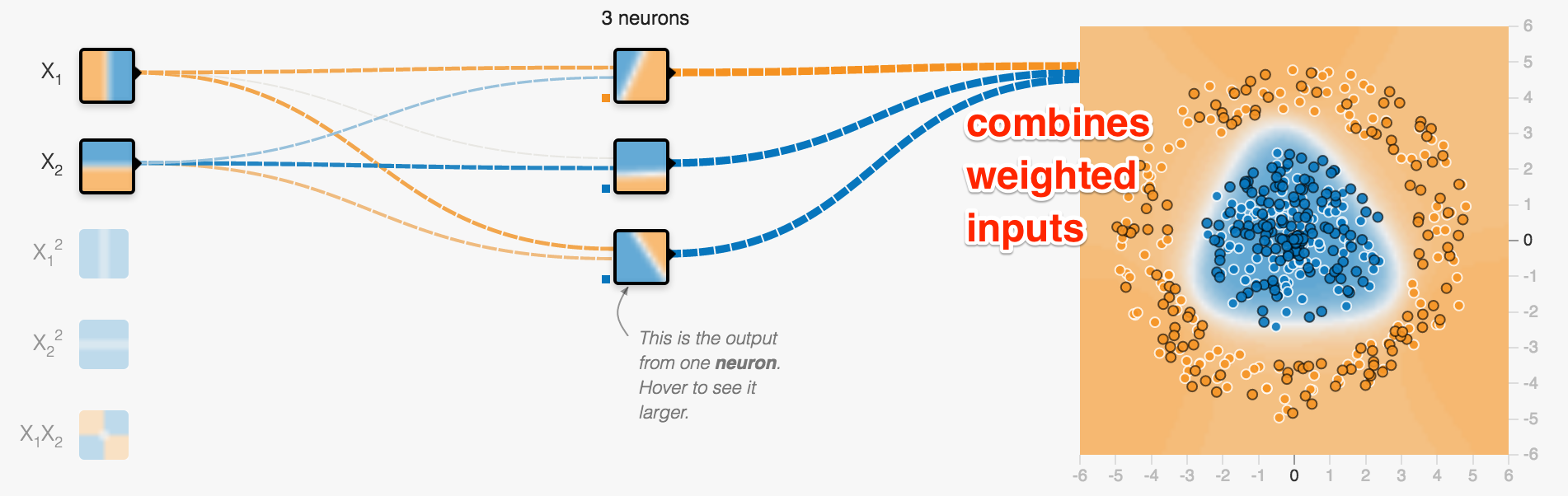again combining all weighted lines to determine two categories: blue or orange?

### Networks can learn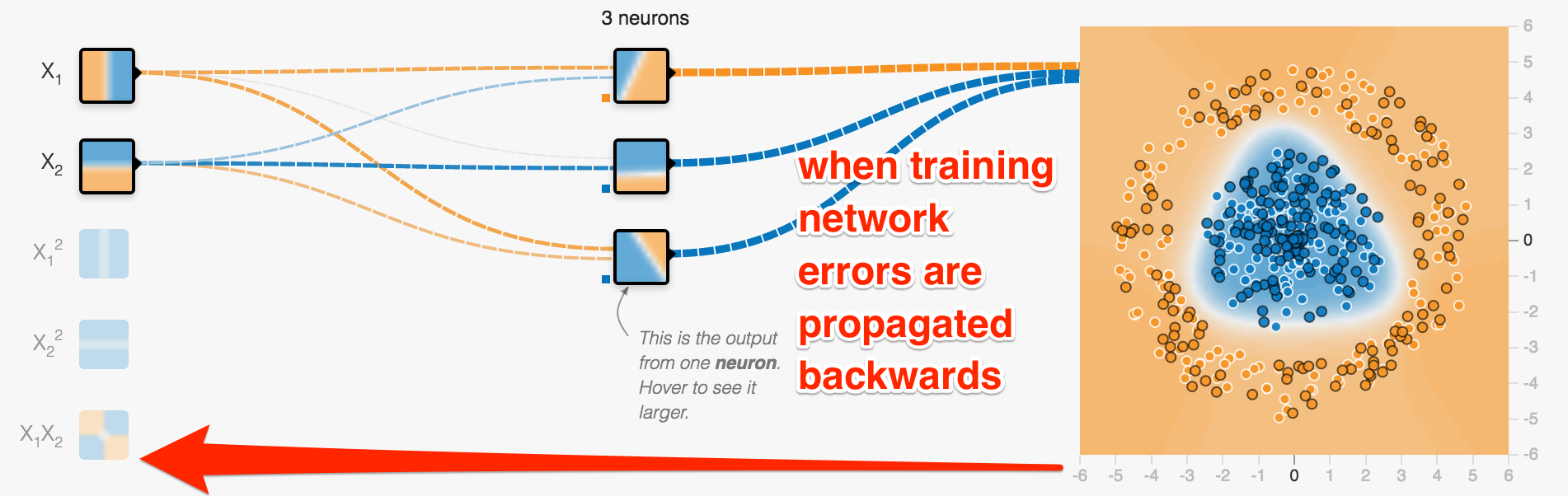• you provide samples and the corresponding right answers
• errors between right answer and prediction are called loss
• they are propagated back to adjust weights of connections
• mathematically an optimisation (often Stochastic Gradient Descent)

### Let's run it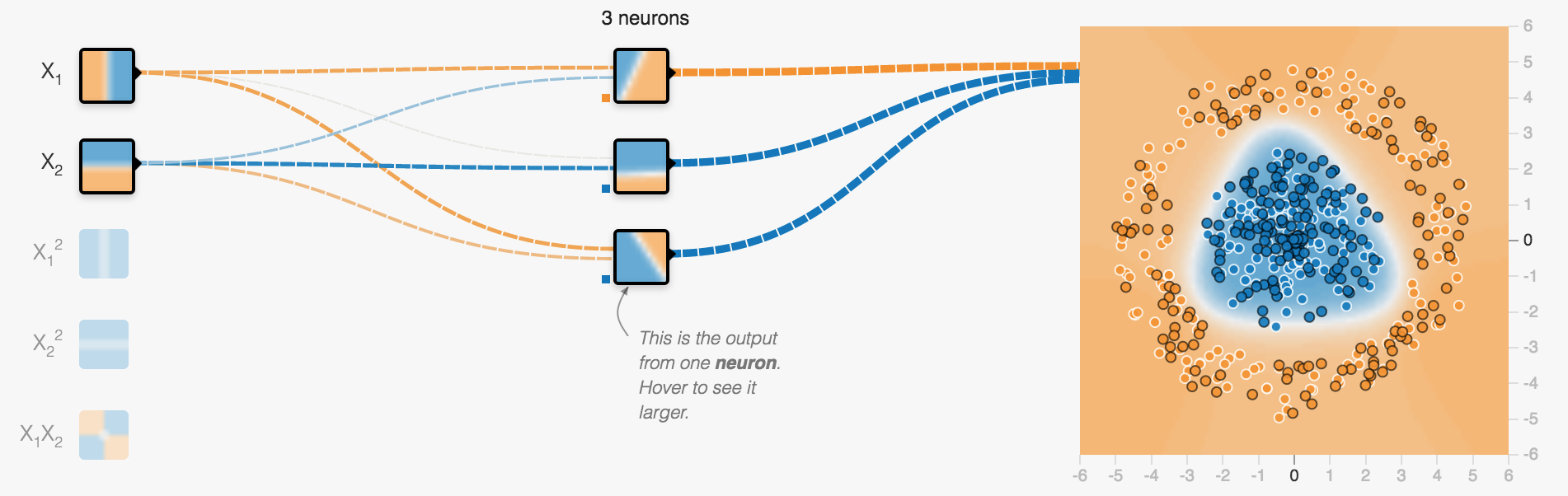### Training and Loss

• data is separated into training and test data
• in image below: training: white border, test: black border
• training data is used to train neural network
• both test and training data are used to check accuracy of prediction
• loss is to be minimized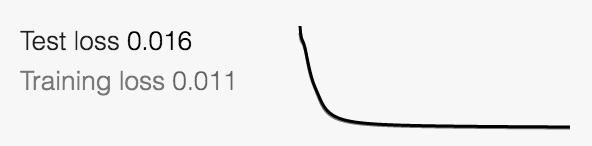### Overfitting

training loss low, test loss much higher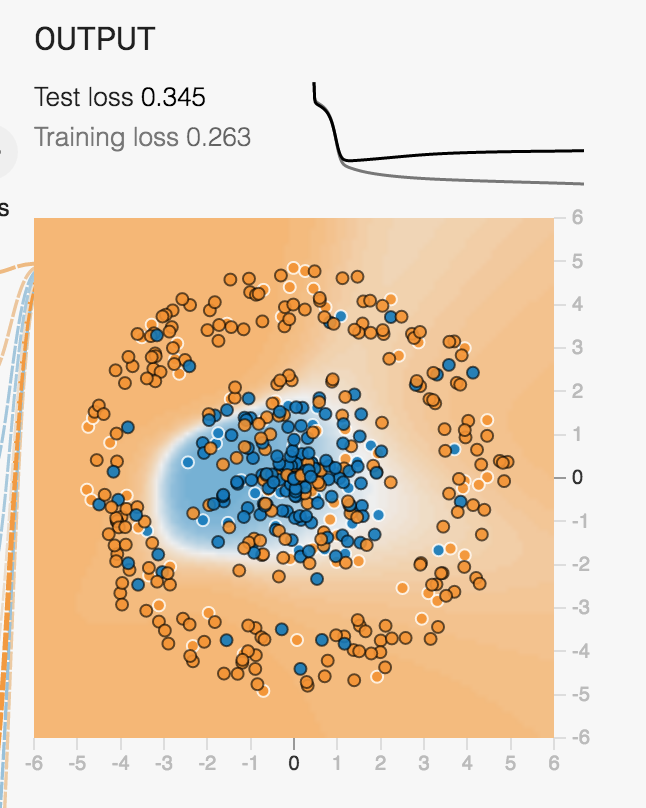NN model is too specific to training values, not general enough

### Deep Neural Networks: More than one hidden layer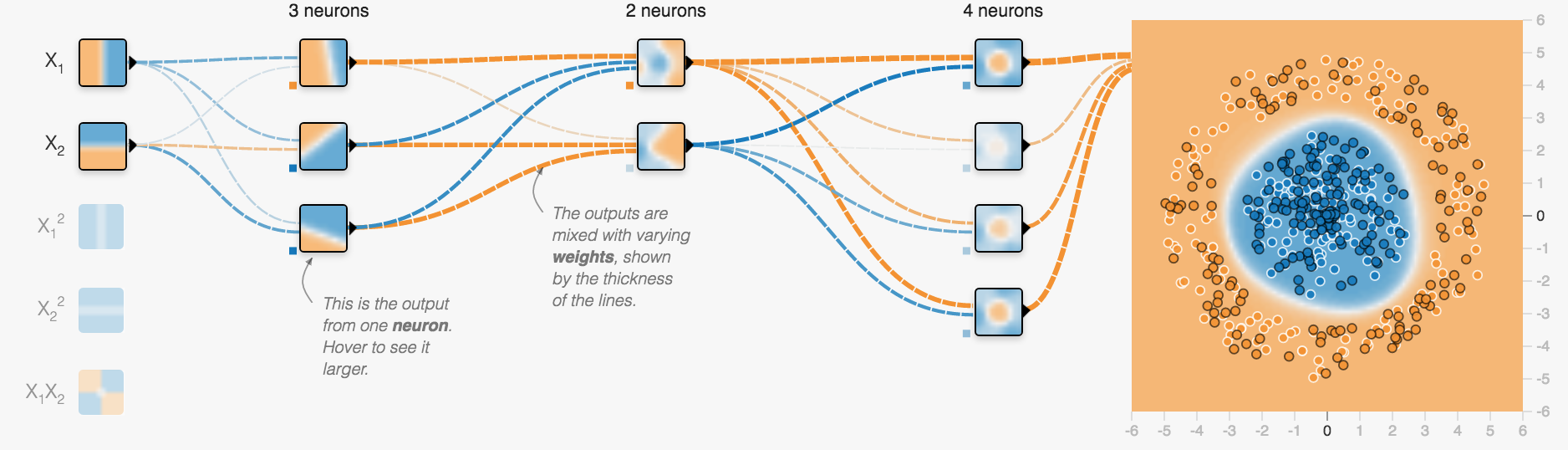each neuron in one layer feeds all neurons in the next layer

## That's it more or less for fully connected feed forward networks

### Main Challenge: What is the best configuration for a given problem?

That means what architecture: How many layers, how many neurons, which activation function?

### Solution: Try it out ...

... using searches over a set of hyper-parameters (might be expensive)

Or: use a pre-trained network (by people who have done that job for you already)

## Convolutional Networks

### Google Inception Convolutional Network architecture to classify images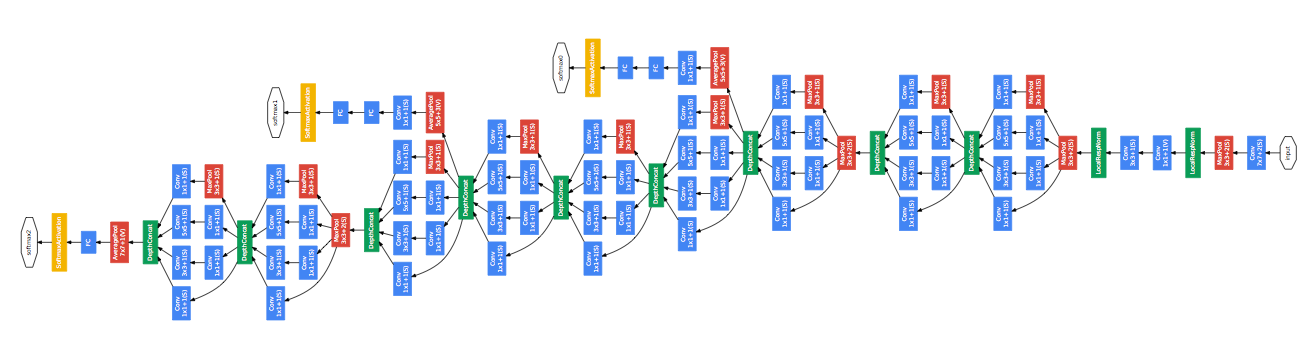Going Deeper with Convolutions

### Intuition for Convolutional Networks

E.g. to recognize dogs (again a classification problem)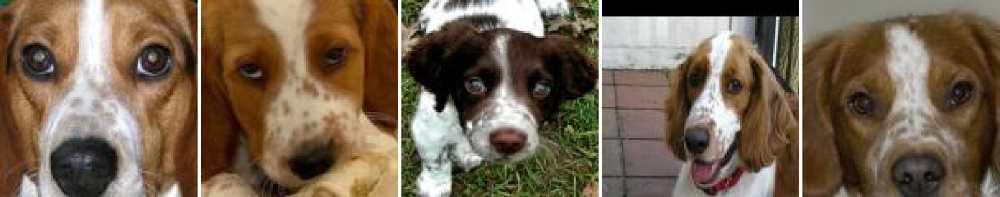using an internal representation like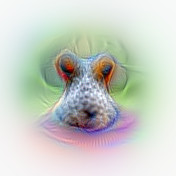https://auduno.github.io/2016/06/18/peeking-inside-convnets/

### Sometimes it is not that easy...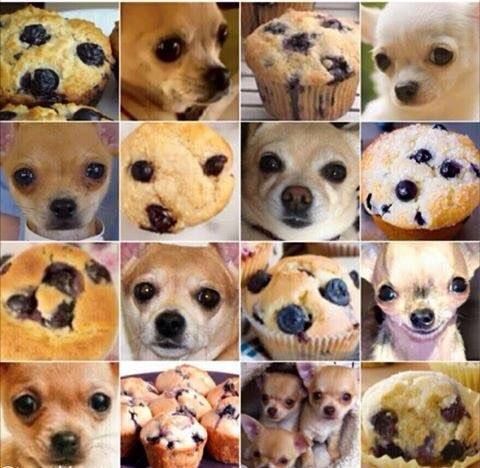Dog vs Muffin

https://twitter.com/mikko/status/751454988631740416?s=03

### TensorFlow finds the Chihuahua!

TensorFlow is the full version of the Playground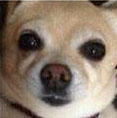``````
Chihuahua (score = 0.68340)
Pomeranian (score = 0.02451)
Pekinese, Pekingese, Peke (score = 0.00751)
toy terrier (score = 0.00716)
beagle (score = 0.00645)
``````

Using the pre-trained Inception model

### It even found out about my secret ...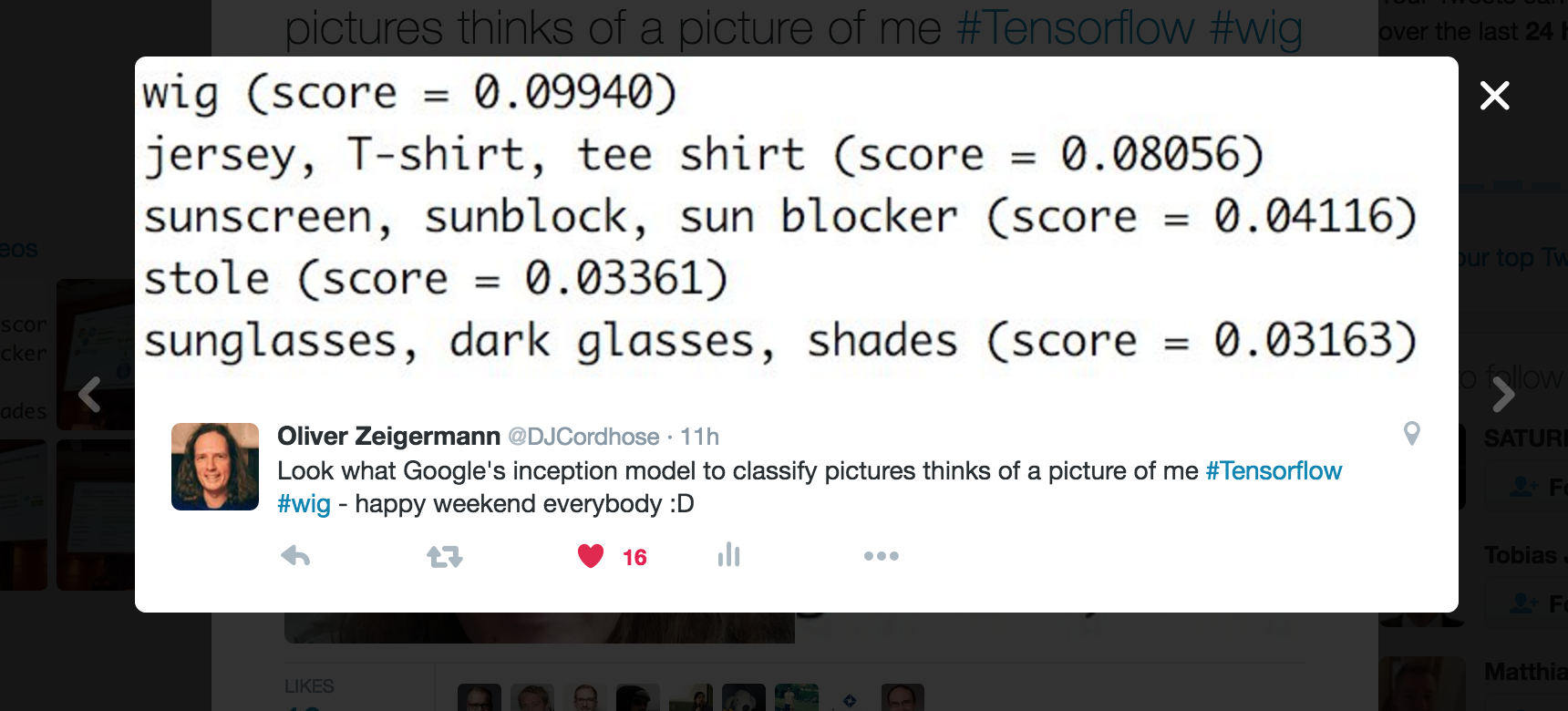https://twitter.com/DJCordhose/status/786875982527467520

## Thank you!

http://bit.ly/ml-js-kongress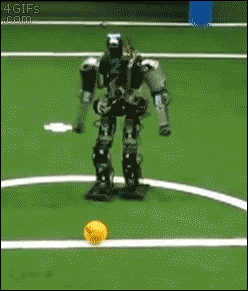No need to be afraid of the Singularity